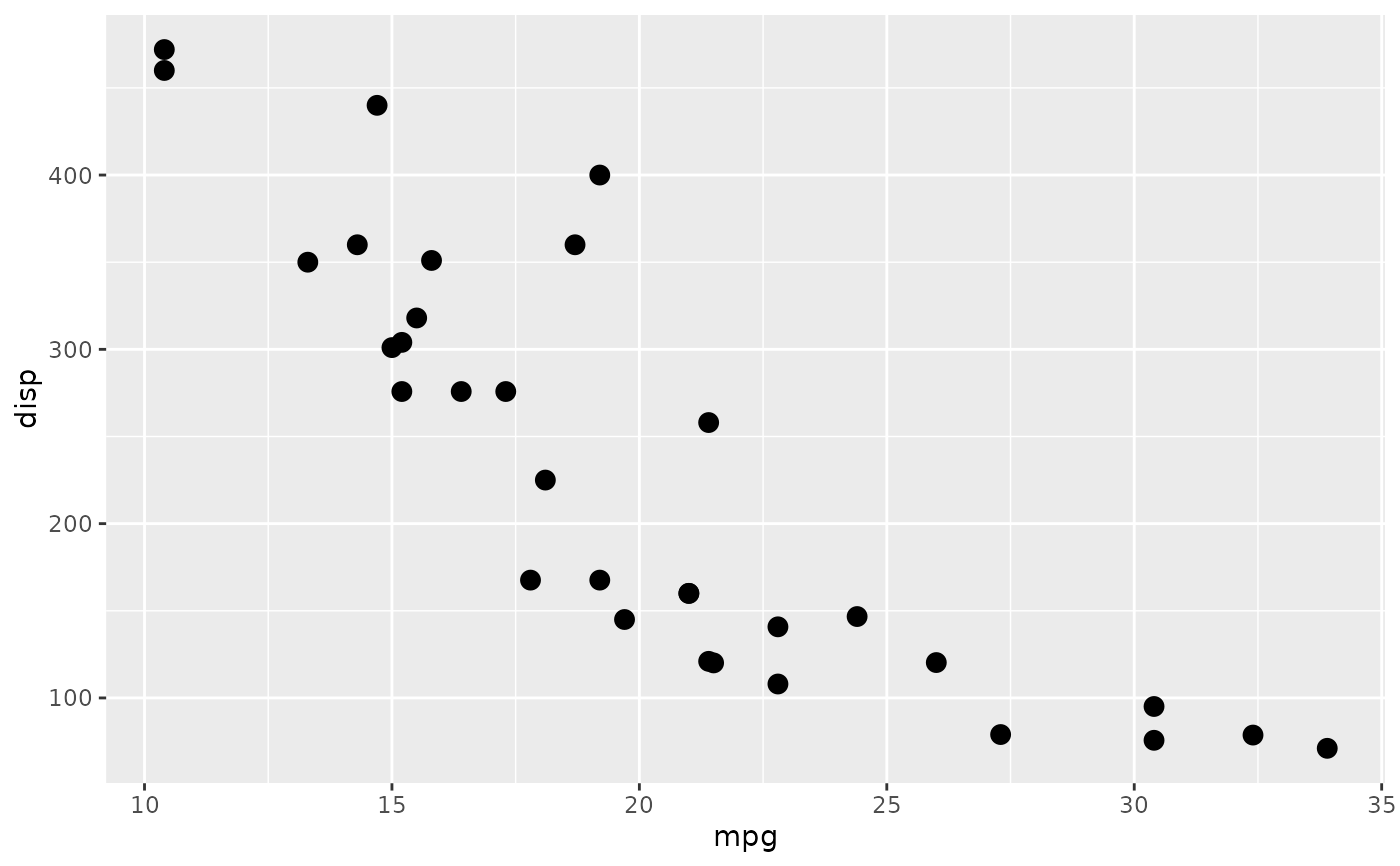This filter adds a directional blur to the provided ggplot layer. The amount of blur, as well as the angle, can be controlled.

with_motion_blur(x, sigma = 0.5, angle = 0, ...)

## Arguments

x

A ggplot2 layer object, a ggplot, a grob, or a character string naming a filter

sigma

The standard deviation of the gaussian kernel. Increase it to apply more blurring. If a numeric it will be interpreted as given in pixels. If a unit object it will automatically be converted to pixels at rendering time

angle

Direction of the movement in degrees (0 corresponds to a left-to-right motion and the angles move in clockwise direction)

...

Arguments to be passed on to methods. See the documentation of supported object for a description of object specific arguments.

## Value

Depending on the input, either a grob, Layer, list of Layers, guide, or element object. Assume the output can be used in the same context as the input.

Other blur filters: with_blur(), with_variable_blur()

## Examples

library(ggplot2)
ggplot(mtcars, aes(mpg, disp)) +
with_motion_blur(
geom_point(size = 3),
sigma = 6,
angle = -45
)